Radiative fluxes are defined with the sign convention that an upward directed flux is a positive quantity.

## Shortwave

The prescription of shortwave radiation follows that of Parkinson and Washington (1979) and Zillman (1972).

For the ocean surface, the net downward shortwave radiation (W/m2) is modeled as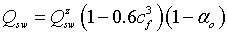For the ice (snow) surface, the net downward shortwave radiation (W/m2) is modeled in an analogous manner as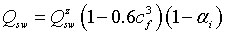The amount of shortwave radiation, prior to its modification by cloud cover and surface albedo effects as formulated above, is the Zillman relation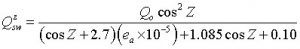where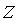is the solar zenith angle, whose cosine is calculated by the geometric formula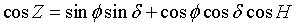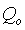is the solar constant, and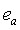is the vapor pressure of water in air.

In the above formula for shortwave radiation,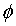is the geographic latitude, and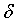is the declination as determined by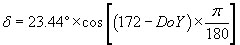where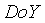is the day-of-year, expressed in 365-day format.

Care has to be taken for solar zenith angles outside the range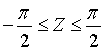that the solar radiation is set to zero

(i.e., the sun is below the local horizon in such cases).

Finally,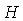is the hour angle given by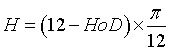where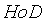is the hour-of-day, expressed in 24-hour format.

The above parameterization of shortwave radiation explicitly accounts for the diurnal cycle of radiation. Models with time steps in excess of 1 hour or so are to be careful that their representation of shortwave radiation is meaningful. A suggestion for such models is to simply eliminate any attempt at resolving the diurnal cycle by instead taking the daily averaged shortwave radiation.

All angles in the above formulae are expressed in radians.

## Longwave

For the ocean surface, the net upward longwave radiation (W/m2) is modeled (Rosati and Miyakoda, 1988)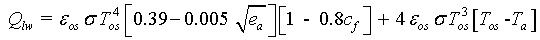For the ice (snow) surface, the net upward longwave radiation (W/m2) is modeled in an analogous manner as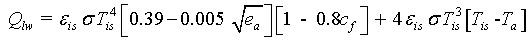NOTE: All temperatures involved in longwave calculations are in Kelvin.